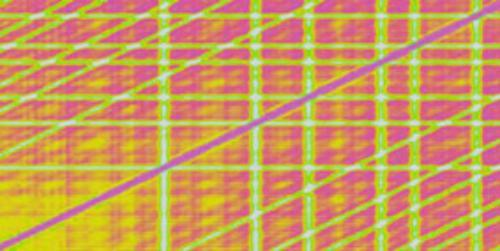# Quantum physics sheds light on Riemann hypothesis

The Riemann Hypothesis is widely regarded as the most important unsolved problem in mathematics. Put forward by Bernhard Riemann in 1859, it concerns the positions of the zeros of the Riemann zeta function in the complex plane.

The Riemann zeta function can be thought of as describing a landscape with the positions of the zeros as features of the landscape. The hypothesis is that, apart from some trivial exceptions, all of the zeros of this function lie on a straight line (known as the critical line).

This is of central importance in mathematics because the Riemann zeta function encodes information about the prime numbers — the atoms of arithmetic. The nontrivial zeros play a central role in an exact formula, first written down by Riemann, for the number of primes in a given range (say, between one and 10 billion). Individual zeros determine correlations between the positions of the primes.

The Riemann zeta function encodes information about the prime numbers —the atoms of arithmetic and critical to modern cryptography on which e-commerce is built.

Finding a proof has been the holy grail of number theory since Riemann first published his hypothesis. It was identified by Hilbert in 1900 as one of his 23 mathematical challenges for the 20th Century, and by the Clay Mathematics Institute in 2000 as one of its seven \$1million Millennium Prize Problems.

At present, the most we know is that at least 40 percent of the (infinitely many) nontrivial zeros satisfy it, and that it is true for the first 100 billion of them.

The University of Bristol has been at the forefront of showing that there are striking similarities between the Riemann zeros and the quantum energy levels of classically chaotic systems.

From a conference in 1996 in Seattle, aimed at fostering collaboration between physicists and number theorists, came early evidence of correlation between the arrangement of the Riemann zeroes and the energy levels of quantum chaotic systems. If this were true it would prove the Riemann hypothesis.

Now, there are certain attributes of the Riemann zeta function called its moments which should give rise to a sequence of numbers. However, before the Seattle conference, only two of these moments were known: 1, as calculated by Hardy and Littlewood in 1918; and 2, calculated by Ingham in 1926.

The next number in the series was suggested as 42 by Conrey (now also at Bristol) and Ghosh in 1992.

The challenge for the quantum physicists then, was to use their quantum methods to check the number 42 and to calculate further moments in the series, while the number theorists tried to do the same using their methods.

Prof Jon Keating and Dr Nina Snaith at Bristol describe the energy levels in quantum systems using random matrix theory. Using RMT methods they produced a formula for calculating all of the moments of the Riemann zeta function. This formula confirmed the number 42.

Two years after Seattle, Keating and Snaith attended a follow-up conference at the Schrodinger Institute in Vienna to present their formula. Meanwhile, number theorists Conrey and Gonek had suggested the next moment in the series.

When Keating and Snaith's formula was used to calculate this moment, it coincided with the number theorists' suggestion: 24,024. The formula really works.

Usually pure mathematics supports physics, supplying the mathematical tools with which physical systems are analysed, but this is a case of the reverse: quantum physics is leading to new insights into number theory.

There has been considerable excitement about these connections between the Riemann Hypothesis and quantum mechanics, but although they have inspired several new lines of attack, the problem still continues to resist. We don't really know why RMT methods work in calculating the moments of the Riemann zeta function. However, they have been used to suggest answers to some other long-standing and important problems relating to the zeta function.

## Random matrix theory and Riemann

The University of Bristol has been at the forefront of showing that there are striking similarities between the Riemann zeros and the quantum energy levels of classically chaotic systems.

Jon Keating and Nina Snaith at Bristol describe the energy levels in quantum systems with random matrix theory. Using RMT methods they produced a formula for predicting all of the moments of the Riemann zeta function.Distribution of complex numbers from the Riemann zeta function imposed on a 2D plot

## Riemann zeta function

The Riemann zeta function encodes information about the prime numbers —the atoms of arithmetic and critical to modern cryptography on which e-commerce is built.

## So why is this work so important?

As we have said, prime numbers are the basic building blocks of mathematics. And primes are vital to cryptography and therefore to the ever-burgeoning world of online commerce and security.